CATEGORIES:

# Ellipse

Definition. An ellipse is the locus of points for which the sum of distances to two fixed points is constant and equal to 2а.

Take two fixed points at a distance 2c apart, join them by a straight line, and extend this line to the x-axis. We draw the perpendicular line through the center of the segment between the focuses and take it for one coordinate axis.

Let us derive the equation of the ellipse.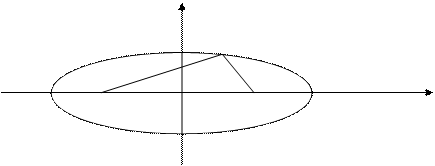у

M4(0;b)

r1 М(х;у)

r2

M1(–a;0) F1 (–c;0) 0 F2 (c;0) M2(a;0) х

M3(0;–b)

The points F1 and F2 are called the foci of the ellipse, and r1 and r2 are its focal radii.

To derive the equation of an ellipse, we take an arbitrary point М(х,у) and consider the distances to the foci: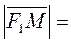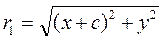,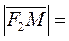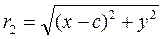.

The characteristic feature of this line is, by definition,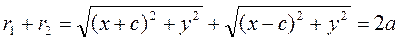.

This is the equation of the ellipse. Let us reduce it to a convenient form: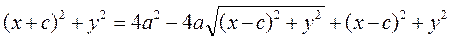,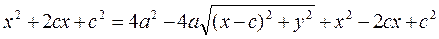.

Eliminating some terms and reducing by 4, we obtain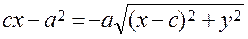.

Let us square both sides:We obtain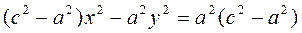.

Let us divide both sides by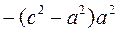: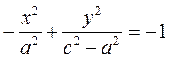;

changing the sign, we obtain the equation of the ellipse:Since the length 2a of a polygonal line is larger than the length 2c of a straight line, we can denote the difference of squares by. (*)

Thus, we obtain the classical equation of an ellipse:. (17)

Date: 2015-01-02; view: 1122

doclecture.net - lectures - 2014-2023 year. Copyright infringement or personal data (0.006 sec.)